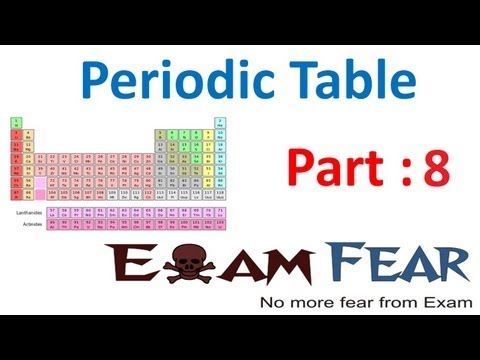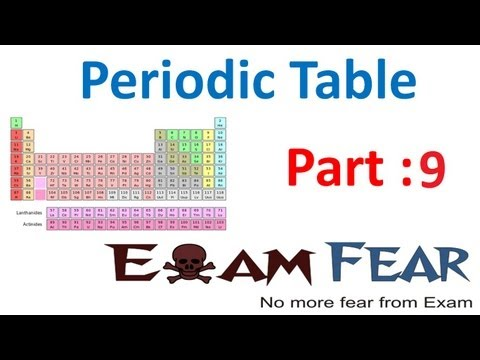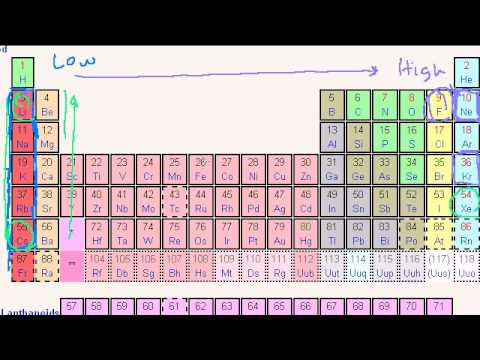## Characteristics of a Period

Subject: Science

#### Overview

The number of valence electrons in a period increase from 1 to 8 on going from left to right in a period. This note describes about variation in various properties of a period in a periodic table.
##### Characteristics of a Period

Characteristics of periods

1. Valence electrons: The number of valence electrons in a period increase from 1 to 8 on going from left to right in a period. It is so because the atomic number of the elements increase by 1 in comparison to the preceding element on going from left to right.
2. Valency: Valency also varies on going from left to right in a period. The valencies of group IA, II A and III A are +1, +2 and +3 respectively but the valencies of group VA, VIA and VII A are -3, -2 and -1 respectively. The valency of group IVA is =4 or -4. And group Zero has no valency.
3. Atomic size: On going from left to right in a period, atomic size decreases. It is so because along with electron, the number of proton in nucleus also increases on going from left to right which holds the outer electron so forcibly which decreases atomic size.
4. Ionization energy:On going from left to right in a period,theionization energy increases as the outer electrons are held tightly by nucleus on going from left to right.5. Metallic characters: The metallic characters decreases on going from left to right.6. Electropositivity: The capacity of losing electrons decreases on going from left to right in a period. So, the electropositivity also decreases on going from left to right.
7. Electronegativity: The capacity of gaining electrons increases as we go from left to right in a period. So, the electronegativity also increases as we go from left to right.### Electronic configuration

The systematic distribution of electrons in different shells of an atom is known as electronic configuration.• 2n2 rule explains the distribution of electrons in different shells of an atom.
• The shells of an atom are named as K, L, M and N.
• According to 2n2 rule, for K shelln=1, so, 2×12 = 2So, K-shell can hold 2 electrons
• For L shell, n=2, so 2×22=8, so, it can hold 8 electrons.
• For M shell, n=3, so 2×32=18, so, it can hold 18 electrons.
• FOR N shell, n=4, so,2×42=32, so it can hold 32 electrons.

But, according to duplet and octet rule, K-shell can hold maximum 2 electrons and the other shells can hold 8 electrons. So, to overcome the contradiction, between 2n2rule and duplet and octet rule, the concept of sub shell is introduced.

### Concept of sub shell

According to this concept, different shell has their sub shell named as s, p, d and f sub shell and they can hold 2,6,10 and 14 electrons respectively.

 Shell Sub Shell No of electron K s 2 L sp 2+6=8 M spd 2+6+10=18 N spdf 2+6+10+14=32##### Things to remember
• Valence electrons, valency, atomic size, ionization of energy, metallic characters, electropositivity, etc. are the characteristics of periods.
• The number of valence electrons in a period increase from 1 to 8 on going from left to right in a period.
• The systematic distribution of electrons in different shells of an atom is known as electronic configuration.
• The capacity of losing electrons decreases on going from left to right in a period. So, the electropositivity also decreases on going from left to right.
• The capacity of gaining electrons increases as we go from left to right in a period. So, the electronegativity also increases as we go from left to right.
• It includes every relationship which established among the people.
• There can be more than one community in a society. Community smaller than society.
• It is a network of social relationships which cannot see or touched.
• common interests and common objectives are not necessary for society.
##### Videos for Characteristics of a Period##### Chemistry Periodicity in properties (Period from electronic configuration)##### Chemistry Periodicity in properties (Periods in periodic table)